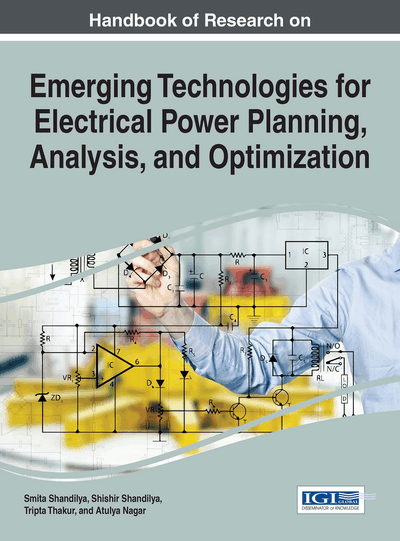# Application of Soft Computing Techniques in Power Engineering

Vivek Venkobarao (IEEE Senior Member, India)
DOI: 10.4018/978-1-4666-9911-3.ch018
Available
\$37.50
No Current Special Offers

## Abstract

A discharge estimator is designed and analyzed in this chapter to estimate the discharge with voltage, frequency and current without prior knowledge of head. A control of discharge in submersible pump is achieved by varying the motor speed. In VSI fed induction motor the speed is varied by varying the switching frequency. The ratio of frequency to the applied voltage is maintained constant, to keep the air gap flux at a desired value. The development of discharge estimator, PID and Fuzzy controllers are discussed in this chapter.
Chapter Preview
Top

## 1. Introduction

The discharge and head of submersible pump are difficult to monitor and record under real-time conditions. So a discharge estimator, which will estimate the discharge close to actual value, is needed. This is achieved by designing a discharge estimator using ANN. In the proposed estimator, discharge is considered as a function of motor input voltage, stator current and frequency. Figure 1 shows the block diagram of the proposed estimator.

The ANN techniques available in MATLAB are used in implementation of the estimator as shown in Figure 2. The training data for the neural network is obtained from the VSI fed induction motor driven submersible pump. The input and output training sets are normalized and the ANN is trained with this normalized data. The estimator developed is tested for various realistic values of inputs and the discharge thus obtained is compared with the simulated results as shown in Figure 3. It can be seen that the error is less than 2%.

Hence this estimator can be used to sense discharge effectively within ± 2% error.

Top

## 2. Discharge Controllers

The function of discharge estimator with the controller is to control discharge without monitoring it. The discharge control is achieved using simple closed loop V/f control. The power circuit consists of uncontrolled rectifier with three-phase ac supply, filter and a PWM inverter. The speed command corresponding to the required discharge is predicted using the block diagram given in Section 4 and is compared with the actual speed command, which is equivalent to the actual discharge. The error is fed to the PID controller and which generates a control signal. The control signal is added with the actual speed signal to generate a frequency command. The frequency command also generates the voltage command through a V/f map. The frequency command and the voltage command are fed to the PWM inverter fed induction motor in order to achieve the required discharge.

Modeling of discharge estimator and PWM inverter as sub blocks for closed loop control to implement maximum efficiency control and constant flow control are given in Figures 4 and 5 respectively. The block diagram includes reference signal generator and current limiter, which are discussed in next section.

Top

## 4. Design Of Reference Signal Estimator

The reference signal estimator generates reference discharge, which can be either for maximum efficiency or constant discharge operations based on the application.

## Complete Chapter List

Search this Book:
Reset